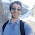## Sunday, July 14, 2019

### Two infinite hat problem and a question about what is well known''

This is a joint post with David Marcus. You will see how he is involved in my next post.

Two infinite hat problems based on one scenario. I am also curious if they are well known.

1) There are an infinite number of people, numbered 1,2,3,... There are 2 colors of hats. They can all see everyone's hat but their own.

2) The adversary is going to put hats on all the people. They will guess their own hat color at the same time.

3) The people can discuss strategy ahead of time, but must use a deterministic strategy and the adversary knows the strategy.

4) The people want to minimize how many they get wrong.

5) The adversary puts on hats to maximize how many they get wrong.

I ask two questions and one meta-question:

Q1: Is there a solution where they get all but a finite number of the guesses right? (I have blogged about a variant of this one a while back.)

Q2: Is there a solution where they get all but at most (say) 18 wrong. (My students would say the answer has to be YES or he wouldn't ask it. They don't realize that I work on upper AND lower bounds!)

Q3: How well known is problem Q1 and the solution? Q2 and the solution? I've seen Q1 and its solution around (not sure where), but the only source on Q2 that I know of is CAN'T TELL YOU IN THIS POST, WILL IN THE NEXT POST. So, please leave a comment telling me if you have seen Q1 or Q2 and solutions. And if so then where.

Feel free to leave any comments you want; however, I warn readers who want to solve it themselves to not look at the comments, or at my next post.

1.Here is one source for Q1 + solution : https://divisbyzero.com/2009/08/11/infinite-hat-problems-solutions/

2.For Q1, I've seen it in a variety of places. For example: https://en.wikipedia.org/wiki/Hat_puzzle#Countably_Infinite-Hat_Variant_without_Hearing.

3.Q1 was common knowledge among uc Berkeley logic students.

Q2 seems to fail by a kind of direct forcing/diagnolization argument. The intuition being the only free bits the players have to communicate not controlled by opponent occur when they get something wrong and you can consider conditions which ensure all your strategies at enemy ensure a certain initial segment but don’t have time to develop now.

1.Q2 is the one I think is not well known.
Can you make your argument that Q2 can't be done rigorous?
If so then email me privately to discuss.
gasarch@cs.umd.edu

4.I am familiar with a slightly different version of #1 (you can only see in one direction), and believe I first encountered it while a student in the Program in Mathematics for Young Scientists (PROMYS), a high-school math camp that teaches proof based mathematics with a focus on number theory. I've never seen the second.

5.As other comments have mentioned, Q1 is a fairly well-known. Q2 I have never heard of.

I claim that Q2 can't be done. Since the adversary knows the strategy, he also knows, given his coloring of the people, what class representative they will choose to guess. The adversary can make the coloring have an arbitrary number of differences with said representative, although still finite.

6.Your argument, if correct and rigorous, would only show that no strategy based on equiv classes and reps can have a bounded number of mistakes. You need to prove that NO strategy can have a bounded number of mistakes.

7.I haven't heard of Q2 before but I imagine that one can argue that the prisoners cannot win as follows.

Look at the finite case: we have n prisoners and they make at most M(n) mistakes; prove that M(n) is unbounded.

Let B={0,1}. The strategy of the n prisoners is the functions f_1,...,f_n, where f_i : B^{n-1} -> B. The strategy of the warden is an element of B^n. Suppose that the prisoners make at most c mistakes. Then for all x element of B^n, in the system of equations S(x) defined

f_i(x[-i]) = x_i, 1≤i≤n

at least n-c are true. (where x_i is the i-th letter and x[-i] is the word x without the i-th letter) This system of equations just says that the guesses are all correct for hat configuration x. But for any fixed i, f_i... equation will be true in only half the systems S(x). So the c holes can be filled by pidgeons quickly.

8.I agree with Edon that it is enough to show that it is not possible in the finite case (because a strategy for the infinite case yields a strategy for every finite case). Another way to state Edon's argument for the finite case was pointed out to me at lunch yesterday: fix a strategy for the case where there are n prisoners. Every prisoner makes a mistake in half of the length n sequences. So the average number of mistakes made per sequence is 2^{n-1}. Thus for some sequence, half the prisoners make mistakes. In other words, M(n) (as defined in Edon's comment) is at least 2^{n - 1}

9.As a follow-up to my previous comment, the argument that I mentioned for the finite case can be adapted to show that Q1 only has a solution if there is a non-Lebesgue measurable set of real numbers (basically the argument is that if every set of real numbers is Lebesgue measurable then the averaging argument still works in the infinite case).

This seems to indicate that a positive answer to Q1 requires some amount of choice. However note that ZF + "every set of reals is Lebesgue measurable" is equiconsistent with ZFC + "there is an inaccessible cardinal". I don't know of a way to show that the consistency of ZFC implies the consistency of ZF + "the answer to Q1 is no" although I suspect that it's true.